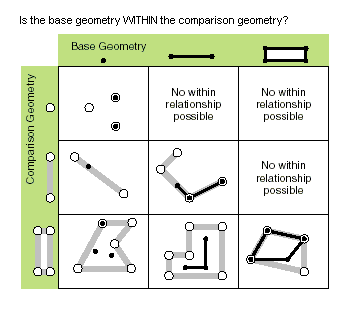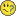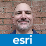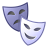# How to use the geometry within function in arcpy?

4266
8
07-11-2017 02:48 PMby Anonymous User
Not applicable

Branched to a new question from: How to use ArcPy Geometry MeasureOnLine

Hey do you know how you would invoke the 'within' method? Been struggling with that one.

It's on the Geometry site, but, alas no good sample 😕 Geometry—Help | ArcGIS for Desktop

Tags (4)
8 RepliesbyEsri Esteemed Contributor

Hi Andrew Valenski , as the help states you have a base geometry which is compared to a comparison geometry to see if the base geometry is "within" the comparison geometry:

boolean_result = base_geometry.within(comparison_geometry)Some examples:

``````def main():
import arcpy
sr = arcpy.SpatialReference(4326)

# create polygon 2 x 2 with LL corner at 0,0
coords = [[0, 0], [0, 2], [2, 2], [2, 0], [0, 0]]
polygon = arcpy.Polygon(arcpy.Array([arcpy.Point(*a) for a in coords]), sr)
print "polygon area:", polygon.area

# create point inside polygon and test within
point = arcpy.PointGeometry(arcpy.Point(1, 1), sr)
print "point within polygon:", point.within(polygon)

# create point at border of polygon
point = arcpy.PointGeometry(arcpy.Point(0, 1), sr)
print "point at border within polygon:", point.within(polygon)

# create polyline inside polygon
line_coords = [[0.5, 0.5], [1.5, 1.5]]
polyline = arcpy.Polyline(arcpy.Array([arcpy.Point(*a) for a in line_coords]), sr)
print "polyline within polygon:", polyline.within(polygon)

# create polyline part of border of polygon
line_coords = coords[1:-1]
polyline = arcpy.Polyline(arcpy.Array([arcpy.Point(*a) for a in line_coords]), sr)
print "polyline on border within polygon:", polyline.within(polygon)

# create polyline that is within polygon but ends on border of polygon
line_coords = [[0.5, 0.5], [1.5, 1.5], [2, 2]]
polyline = arcpy.Polyline(arcpy.Array([arcpy.Point(*a) for a in line_coords]), sr)
print "polyline ending on border of polygon within polygon:", polyline.within(polygon)

# create polygon inside larger polygon
coords = [[0.5, 0.5], [0.5, 1], [1, 1], [1, 0.5], [0.5, 0.5]]
polygon2 = arcpy.Polygon(arcpy.Array([arcpy.Point(*a) for a in coords]), sr)
print "small polygon within larger polygon:", polygon2.within(polygon)

# create polygon with a single point on border of larger polygon
coords = [[0, 0], [0.5, 1], [1, 1], [1, 0.5], [0, 0]]
polygon2 = arcpy.Polygon(arcpy.Array([arcpy.Point(*a) for a in coords]), sr)
print "small polygon with a single point on border of larger polygon is within larger polygon:", polygon2.within(polygon)

# create polygon thar shares and edge with larger polygon
coords = [[0, 0], [0, 2], [1, 1], [0, 0]]
polygon2 = arcpy.Polygon(arcpy.Array([arcpy.Point(*a) for a in coords]), sr)
print "small polygon which shares edge of larger polygon is within larger polygon:", polygon2.within(polygon)

if __name__ == '__main__':
main()‍‍‍‍‍‍‍‍‍‍‍‍‍‍‍‍‍‍‍‍‍‍‍‍‍‍‍‍‍‍‍‍‍‍‍‍‍‍‍‍‍‍‍‍‍‍‍‍‍‍``````

The results are:

``````polygon area: 4.0
point within polygon: True
point at border within polygon: False
polyline within polygon: True
polyline on border within polygon: False
polyline ending on border of polygon within polygon: True
small polygon within larger polygon: True
small polygon with a single point on border of larger polygon is within larger polygon: True
small polygon which shares edge of larger polygon is within larger polygon: True‍‍‍‍‍‍‍‍‍``````byEsri Esteemed Contributor

``````    # polyline on border of polygon
line_coords = [[0, 2], [2, 2], [2, 0]]
polyline = arcpy.Polyline(arcpy.Array([arcpy.Point(*a) for a in line_coords]), sr)
print "test 1: polyline on border of polygon is within polygon:", polyline.within(polygon)

# polyline that ends on polygon border
line_coords = [[2, 0], [1, 1]]
polyline = arcpy.Polyline(arcpy.Array([arcpy.Point(*a) for a in line_coords]), sr)
print "test 2: polyline that end on border of polygon is within polygon:", polyline.within(polygon)

# polyline that combines line 1 (within is false) and line 2 (within is true)
line_coords = [[0, 2], [2, 2], [2, 0], [1, 1]]
polyline = arcpy.Polyline(arcpy.Array([arcpy.Point(*a) for a in line_coords]), sr)
print "test 3: polyline that combines line 1 (within is False) and line 2 (within is True) is within polygon:", polyline.within(polygon)
‍‍‍‍‍‍‍‍‍‍‍‍‍‍``````

which resulted in...

``````test 1: polyline on border of polygon is within polygon: False
test 2: polyline that end on border of polygon is within polygon: True
test 3: polyline that combines line 1 (within is false) and line 2 (within is true) is within polygon: True‍‍‍``````

So a line on the border of a polygon is not with the polygon, but if I add a single point to the line that is inside the polygon the line suddenly is within the polygon... interesting ...by Anonymous User
Not applicable

xander_bakker‌ This is awesome! Thanks!

Is it also possible to use Feature classes as the base and secondary geometry? I.e. set a point FC as a variable and a polygon as another variable and call:

Pt = "C:\...\Cities"

Pg = "C:\...\Counties"

Pt.within(Pg)

I tried running this in the IDE interface, but got an error. Any ideas?byEsri Esteemed Contributor

So a line on the border of a polygon is not with the polygon, but if I add a single point to the line that is inside the polygon the line suddenly is within the polygon

If you look at the Clementini* rules, this makes perfect sense. A geometry is composed of boundary and interior. The boundary of a line is the start and end points; the boundary of a polygon is its perimeter ring(s) (points don't have interior). So the "within" relationship tests for an intersection of the (line) figure with the (polygon) interior, and "line on boundary" does not intersect the polygon interior (empty set), so it's not "within".

Then there's "Completely Within" and "Contains", and "Contained", but I have a scrum, so I'm not going there...

- V

*Eliseo Clementini, Paolino Di Felice, and Peter van Oosterom, A Small Set of Formal Topological Relationships Suitable for End-User Interaction, Proceedings of the Third International Symposium on Advances in Spatial Databases, p.277-295, June 23-25, 1993byEsri Esteemed Contributor

Hi Vince Angelo , you are absolutely right. Yesterday I read an old 9.3 document where the Clementini rules were explained and the results correspond perfectly to that.  Thanks for the additional information.byMVP Esteemed Contributor

Xander Bakker‌, the screenshot you include at the top of your reply is from ArcGIS 10.3.  The reason I point that out is starting in ArcGIS 10.4.x (I can't remember if it was 10.4 or 10.4.1) Esri added a relation parameter to many of the geometry spatial methods, including within:  Geometry—Help | ArcGIS Desktop (10.4)

`within (second_geometry, {relation})`
 Parameter Explanation Data Type second_geometry A second geometry. Object relation The spatial relationship type.BOUNDARY — Relationship has no restrictions for interiors or boundaries.CLEMENTINI — Interiors of geometries must intersect. Specifying CLEMENTINI is equivalent to specifying None. This is the default.PROPER — Boundaries of geometries must not intersect.(The default value is None) String

Although I am glad the relations were finally added, the default arguments are different between the ArcPy Geometry classes and Select Layer by Location for certain spatial methods, including within.  For the ArcPy Geometry classes, the default relation is CLEMENTINI whereas the default or unqualified within for the Select Layer by Location tool is BOUNDARY.byEsri Esteemed Contributor

Hi bixb0012 , for some reason my help page defaulted to 10.3 (that happens sometimes) and I didn't notice the enhancement. Very useful information and indeed a great addition to have control over the relation. Thanks for sharing!by
New Contributor III

For the ArcPy 'within' method, I'm having a hard time coming up with a scenario where the CLEMENTINI option returns a result different from the PROPER option.

For example: I have a line and a rectangle. The line starts at a vertex of the rectangle and dangles into the interior of the rectangle. The methods line.within(rectangle, 'CLEMENTINI') and line.within(rectangle, 'PROPER') both produce True.

I expect the 'PROPER' option to return False since the end of the line intersects a vertex of the rectangle. As Vince said, the boundary of a line includes its start and end points, and the boundary polygon is its actual boundary. The relationship between these two geometries intersects at a point, so I'd think the 'PROPER' relation paramater would produce a False. At least that's how I read it in the documentation...

Any ideas why these are both True? Let me know if this is worth a separate discussion/question elsewhere in GeoNet.